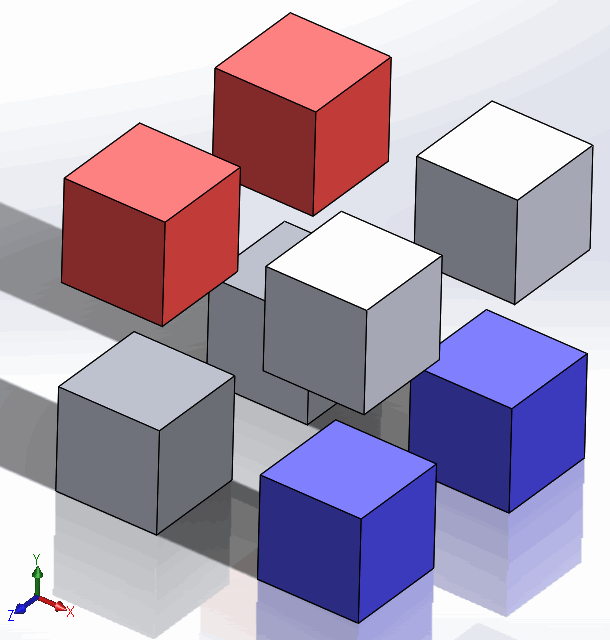# Batch add components and position them in the grid using SOLIDWORKS APIComponents inserted into 2 x 2 x 2 grid

This example demonstrates the performance efficient way of inserting a batch of components into assembly and automatic positioning of them in 3D grid using SOLIDWORKS API.

Components are inserted using the IAssemblyDoc::AddComponents3 SOLIDWORKS API method. Which allows to preassign the transformations for components to be inserted.

Boundaries of the grid can be specified by setting the constants in the begging of the macro.

```Const ROWS_COUNT As Integer = 2 'maximum number of components in a row (parallel to X)
Const COLUMNS_COUNT As Integer = 2 'maximum number of components in a row (parallel to Y)
Const DISTANCE As Double = 0.1 'distance between components in rows, columns and levels
```

Specify the list of components to insert by assigning the values of compsPaths array. Inserting the same component path in different positions is supported.

```Dim compsPaths(N) As String

compsPaths(0) = "Full path to part or assembly"
compsPaths(1) = "Full path to part or assembly"
...
compsPaths(N) = "Full path to part or assembly"
```

```Const ROWS_COUNT As Integer = 2
Const COLUMNS_COUNT As Integer = 2
Const DISTANCE As Double = 0.1

Dim swApp As SldWorks.SldWorks

Sub main()

Set swApp = Application.SldWorks

Dim compsPaths(7) As String

compsPaths(0) = "D:\models\box1.sldprt"
compsPaths(1) = "D:\models\box2.sldprt"
compsPaths(2) = "D:\models\box3.sldprt"
compsPaths(3) = "D:\models\box1.sldprt"
compsPaths(4) = "D:\models\box1.sldprt"
compsPaths(5) = "D:\models\box2.sldprt"
compsPaths(6) = "D:\models\box3.sldprt"
compsPaths(7) = "D:\models\box1.sldprt"

Dim swAssy As SldWorks.AssemblyDoc

Set swAssy = swApp.ActiveDoc

If Not swAssy Is Nothing Then
InsertComponents swAssy, compsPaths, ROWS_COUNT, COLUMNS_COUNT, DISTANCE
Else
MsgBox "Please open assembly"
End If

End Sub

Sub InsertComponents(assy As SldWorks.AssemblyDoc, vPaths As Variant, rows As Integer, columns As Integer, dist As Double)

Dim transforms() As Double
ReDim transforms((UBound(vPaths) + 1) * 16 - 1)

Dim coordSys() As String
ReDim coordSys(UBound(vPaths))

Dim level As Integer
Dim row As Integer
Dim column As Integer

Dim i As Integer

For i = 0 To UBound(vPaths)

If row = rows Then

row = 0
column = column + 1

If column = columns Then
column = 0
level = level + 1
End If

End If

Dim vTransform As Variant
vTransform = ComposeTransform(row * dist, column * dist, level * dist)

Dim j As Integer

For j = 0 To UBound(vTransform)
transforms(i * (UBound(vTransform) + 1) + j) = vTransform(j)
Next

row = row + 1

Next

assy.AddComponents3 vPaths, transforms, coordSys

End Sub

Function ComposeTransform(x As Double, y As Double, z As Double) As Variant

Dim dMatrix(15) As Double
dMatrix(0) = 1: dMatrix(1) = 0: dMatrix(2) = 0: dMatrix(3) = 0
dMatrix(4) = 1: dMatrix(5) = 0: dMatrix(6) = 0: dMatrix(7) = 0
dMatrix(8) = 1: dMatrix(9) = x: dMatrix(10) = y: dMatrix(11) = z
dMatrix(12) = 1: dMatrix(13) = 0: dMatrix(14) = 0: dMatrix(15) = 0

ComposeTransform = dMatrix

End Function
```